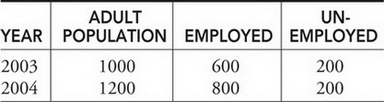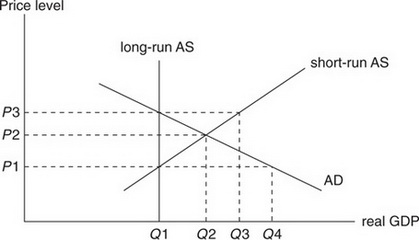# AP Macroeconomics Practice Test 19

### Test Information12 questions14 minutes

1. Which of the following transactions would be included in the official computation of gross domestic product?

2. Theo loses his job at the public swimming pool when the pool closes for the winter. This is an example of

3. Which of the following is not a scarce economic resource?

4. How does an increasing national debt impact the market for U.S. dollars and the value of the dollar with respect to other currencies?

MARKET FOR THE DOLLAR     VALUE OF THE DOLLAR

5. Suppose the price level in the United States has risen in the past year, but production of goods and services has remained constant. Based on this information, which of the following is true?

NOMINAL GDP     REAL GDP

6. Which of the following is not an addition to national income?

7. Which choice produces a faster rate of economic growth for the United States?

8.The table above summarizes the local labor market. Based on this information, which of the following is an accurate statement?

9. Which of the following is true of money and financial markets?

10. Which of the following would increase the aggregate demand function?

11.The figure above shows aggregate demand (AD) and supply (AS) for the economy. Assuming that aggregate demand remains constant, which of the following best predicts the short-run price level, the long-run price level, and the long-run level of output?

SHORT-RUN PRICE LEVEL     LONG-RUN PRICE LEVEL     LONG-RUN OUTPUT

12. Which of the following is not included in the U.S. GDP?